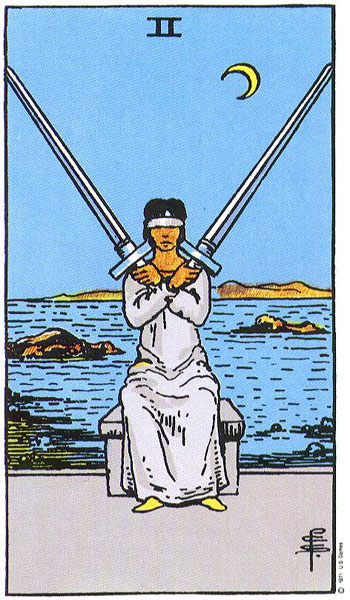## people with a 56 (Two of Swords) life lesson

### December 10, 1970people with a 56 life lessonJacqueline Kennedy Onassis / Jackie O.
born July 28th, 1929

7 + 28 +1+9+2+9 = 56 = her life lesson = what she was here to learn = Decisive.  Choosing.  Selection.  Decisions.  Maybe.  Serenity.  Diplomacy.  Ambassador.  Peacemaking.  Negotiate.  Compromise.  Settlement.  Conciliatory.  Mediate.  Cease fire.  Neutral.  Customer service.  Agreeable.  Agreement.  Accommodate.  Poise.  Composure.  Tact.  Graceful.  Congenial.  Civility.  Libran.  Ambivalent.  Delicate.  Truce.  Treaty.  Accord.  Pact.  Pacify.  Tiebreaker.  Tie.  Stalemate.  A draw.  Standoff.  Non-answers.

Ryan Reynolds

born October 23rd, 1976                           10 + 23 +1+9+7+6 = 56

Jennifer Lopez

born July 24th, 1969                                   7 + 24 +1+9+6+9 = 56

Lil Wayne

born September 27th, 1982                      9 + 27 +1+9+8+2 = 56

Maika Monroe

born May 29th, 1993                                   5 + 29 +1+9+9+3 = 56

Emanuela de Paula

born April 25th, 1989                                 4 + 25 +1+9+8+9 = 56

Al Gore
born March 31st, 1948                                3 + 31 +1+9+4+8 = 56

Peggy Fleming
born July 27th, 1948                                   7 + 27 +1+9+4+8 = 56

born October 30th, 1735                             10 + 30 +1+7+3+5 = 56

Cokie Roberts
born December 27th, 1943                         12 + 27 +1+9+4+3 = 56

Daniel Tosh

born May 29th, 1975                                    5 + 29 +1+9+7+5 = 56

Hayden Panettiere

born August 21st, 1989                               8 + 21 +1+9+8+9 = 56

Michelle Pfeiffer
born April 29th, 1958                                  4 + 29 +1+9+5+8 = 56

LeAnn Rimes
born August 28th, 1982                               8 + 28 +1+9+8+2 = 56

Debbie Gibson
born August 31st, 1970                                8 + 31 +1+9+7+0 = 56

John McCain
born August 29th, 1936                               8 + 29 +1+9+3+6 = 56

Lisa Kudrow
born July 30th, 1963                                     7 + 30 +1+9+6+3 = 56

Louis Tomlinson

born December 24th, 1991                         12 + 24 +1+9+9+1 = 56